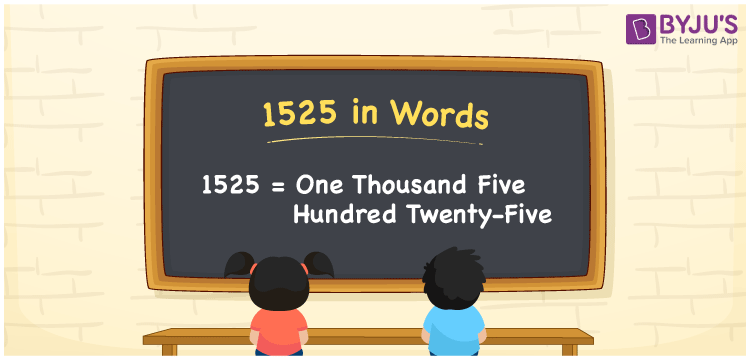# 1525 in Words

We can write 1525 in words as One thousand five hundred twenty-five. If you got a voucher worth Rs. 1525 on purchasing a TV, you can convey the same to others as, “I got a voucher of One thousand five hundred twenty-five on purchase of a TV”. That means we use the word form of the cardinal number 1525 in many chats.

 1525 in words One thousand five hundred twenty-five One thousand five hundred twenty-five in Numbers 1525

## 1525 in English Words

Using the English alphabet, we can write numbers in words. So, we can spell 1525 in English as “One thousand five hundred twenty-five”.## How to Write 1525 in Words?

The conversion of the number 1525 into words is simple when we use the place value chart as given below.

 Thousands Hundreds Tens Ones 1 5 2 5

Here, ones = 5, tens = 2, hundreds = 5, and thousands = 1.

Thus, we can write the expanded form as:

1 × Thousand + 5 × Hundred + 2 × Ten + 5 × One

= 1 × 1000 + 5 × 100 + 2 × 10 + 5 × 1

= 1000 + 500 + 20 + 5

= One thousand + Five hundred + Twenty + Five

= One thousand five hundred twenty-five

Thus, 1525 in words = One thousand five hundred twenty-five.

As we know, 1525 is a natural number that precedes 1526 and succeeds 1524.

1525 in words – One thousand five hundred twenty-five

Is 1525 an odd number? – Yes

Is 1525 an even number? – No

Is 1525 a prime number? – No

Is 1525 a composite number? – Yes

Is 1525 a perfect square number? – No

Is 1525 a perfect cube number? – No

## Frequently Asked Questions on 1525 in Words

Q1

### How do you write 1525 in words?

We can write 1525 in words as One thousand five hundred twenty-five.
Q2

### How to write Rs. 1525 in words on a cheque?

On a cheque, we generally write Rs. 1525 in words as “One thousand five hundred twenty-five rupees only”.
Q3

### Write the value of 1525 + 2975 in words.

1525 + 2975 = 4500 Therefore, the value of 1525 + 2975, i.e., 4500 can be written in words as Four thousand five hundred.